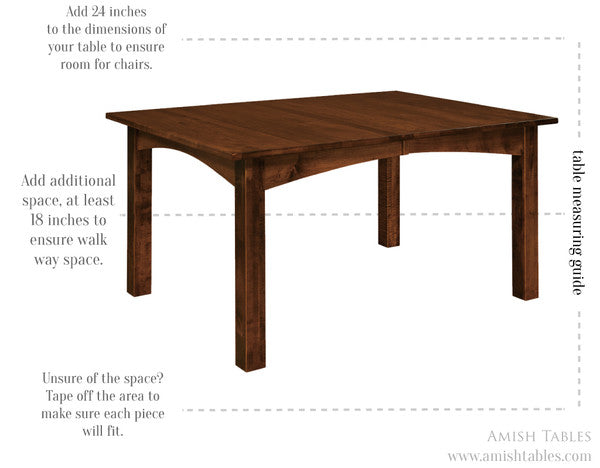Table Seating Measurements

Our seating measurements are based on 24" of space per person.  Below are calculations on how to figure out how many people you can seat at our tables.For Square or Rectangular Tables with Extensions
Add the Table Top size with your number of extensions, then divide that number by 24".  That number is how many people can sit at one side of the table with the extensions.  Multiply that number by 2 and then add 2 more for seating at the ends.
Ex: 72 + 48=120 / 24 = 5 x 2 +2 = 12.  A 72" table with 4 - 12" extensions will seat 12 people.

For Square or Rectangular Tables without Extensions
Divide the Table Top size by 24".  That number is how many people can sit at one side of the table with the extensions.  Multiply that number by 2 and then add 2 more for seating at the ends.
Ex: 72 / 24 = 3 x 2 +2 = 8.  A 72" table will seat 8 people.

For Round Tables
Multiply the table top size by 3.14 and then divide by 24".
Ex: 60 x 3.14 = 188.4 / 24 = 7.85.  A 60" solid top table will seat 7 - 8 people.

For Oval Tables
The length of your table in feet is the number of people you can seat.